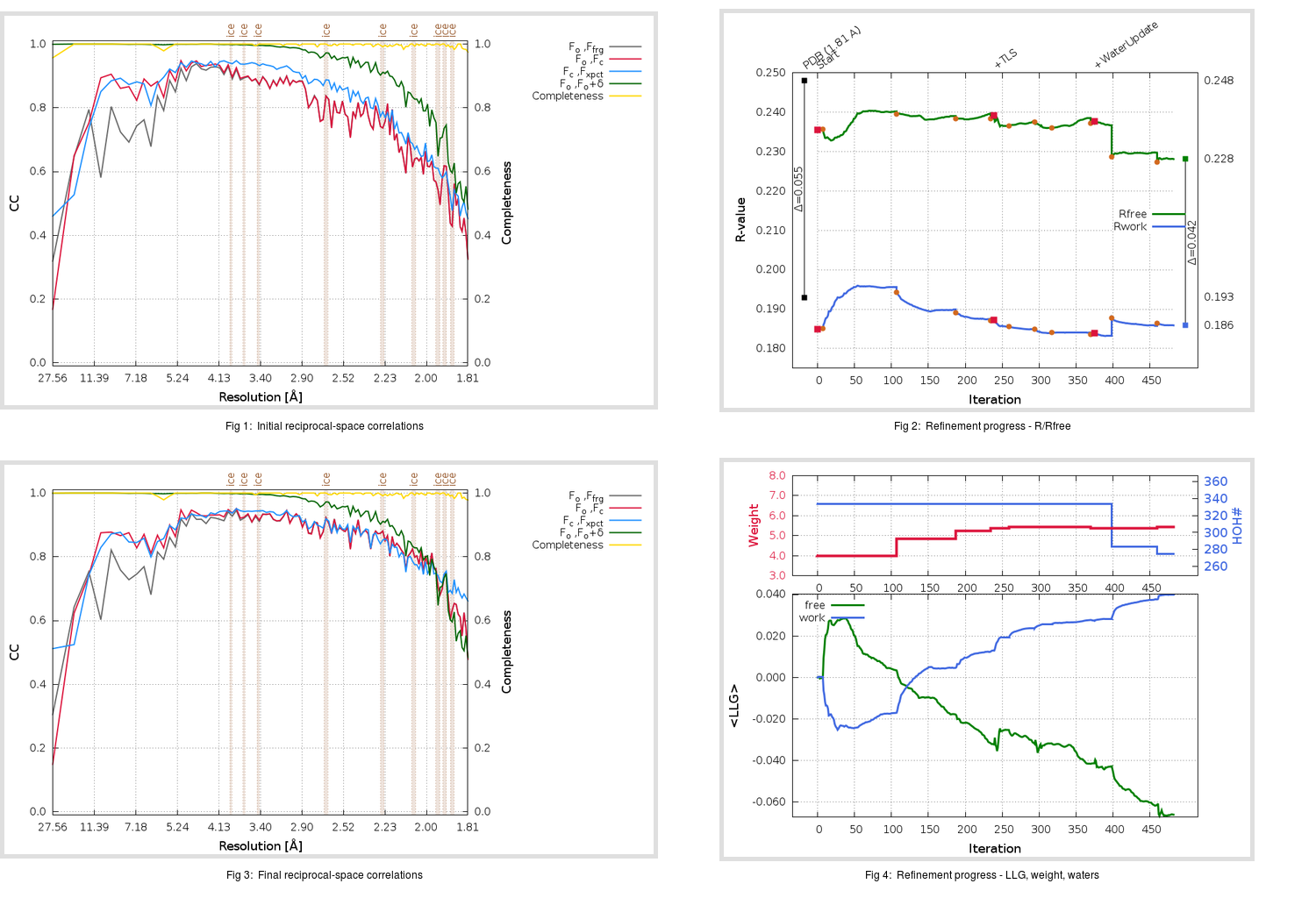Content:

## Deposited

` `
 Date deposited Date data collection Resolution R, Rfree 20200315 20200227 1.81 0.1900 0.2480

Molprobity (CCP4 7.0 version) summary:

```Ramachandran outliers =   0.66 %
favored =  96.03 %
Rotamer outliers      =   0.38 %
C-beta deviations     =     1
Clashscore            =   6.34
RMS(bonds)            =   0.0137
RMS(angles)           =   1.83
MolProbity score      =   1.62
Resolution            =   1.81
R-work                =   0.1900
R-free                =   0.2480
```

```Number of waters      =   334

<B> (all atoms) =   31.92 ( sd =   11.20 ) for       2731 non-hydrogen atoms
<B>   (protein) =   30.35 ( sd =   10.21 ) for       2370 non-hydrogen atoms
<B>     (water) =   42.46 ( sd =   11.46 ) for        334 non-hydrogen atoms
<B>    (others) =   37.68 ( sd =   18.32 ) for         27 non-hydrogen atoms

B min/max       (all non-hydrogen atoms) =   15.13 /  107.70
B min/max   (protein non-hydrogen atoms) =   15.96 /   88.08
B min/max     (water non-hydrogen atoms) =   15.13 /  107.70
B min/max     (other non-hydrogen atoms) =   22.29 /   60.86
```

## BUSTER (re-)refinement

` `

Molprobity (CCP4 7.0 version) summary:

```Ramachandran outliers =   0.33 %
favored =  98.01 %
Rotamer outliers      =   1.14 %
C-beta deviations     =     0
Clashscore            =   3.59
RMS(bonds)            =   0.0116
RMS(angles)           =   1.56
MolProbity score      =   1.19
Resolution            =   1.81
R-work                =   0.1859
R-free                =   0.2281
```

```Number of waters      =   275

<B> (all atoms) =   31.19 ( sd =    9.04 ) for       2672 non-hydrogen atoms
<B>   (protein) =   29.81 ( sd =    7.91 ) for       2370 non-hydrogen atoms
<B>     (water) =   42.15 ( sd =    9.29 ) for        275 non-hydrogen atoms
<B>    (others) =   40.67 ( sd =   19.19 ) for         27 non-hydrogen atoms

B min/max       (all non-hydrogen atoms) =   14.11 /   68.49
B min/max   (protein non-hydrogen atoms) =   14.11 /   68.49
B min/max     (water non-hydrogen atoms) =   15.78 /   67.97
B min/max     (other non-hydrogen atoms) =   28.64 /   62.78
```

Refinement progression:Results:

` `
 File Remark 5RE8_aB_refine.01_03_refine.pdb.gz exact refinement commands are in header 5RE8_aB_refine.01_03_refine.mtz.gz including original deposited data and several re-refinement map coefficients 5RE8_aB_refine.01_03_BUSTER_model.cif.gz including any non-standard compound restraints 5RE8_aB_refine.01_03_BUSTER_refln.cif.gz